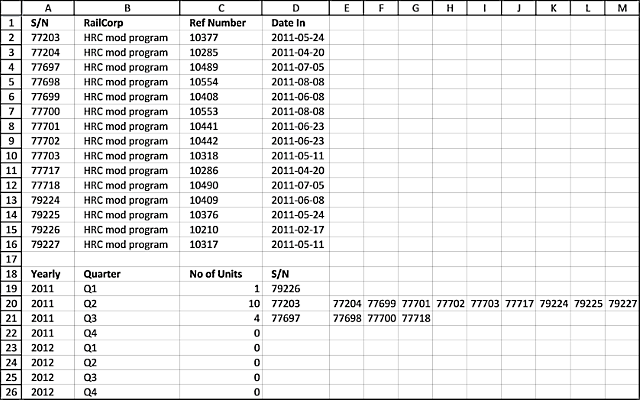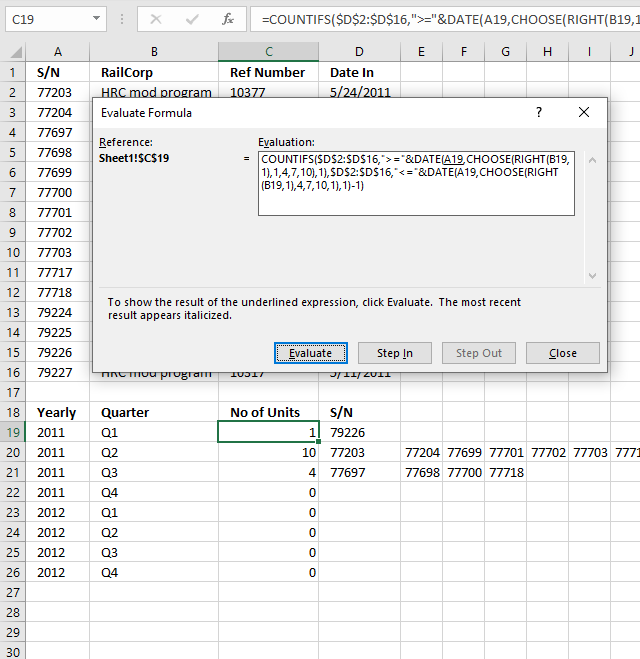Author: Oscar Cronquist Article last updated on February 23, 2021This article demonstrates two formulas, the first formula counts items by quarter and the second formula extracts the corresponding items horizontally.

The image above shows a formula in cell C19 that extracts numbers from column A based on the corresponding date in column D. If the date is in a given year and quarter specified in cell A19 and B19 and cells below the numbers show up in column E and columns to the right as far as needed.

Sam asks:S/N RailCorp Ref Number Date In
77203 HRC mod program 10377 24/05/2011
77204 HRC mod program 10285 20/04/2011
77697 HRC mod program 10489 5/07/2011
77698 HRC mod program 10554 8/08/2011
77699 HRC mod program 10408 8/06/2011
77700 HRC mod program 10553 8/08/2011
77701 HRC mod program 10441 23/06/2011
77702 HRC mod program 10442 23/06/2011
77703 HRC mod program 10318 11/05/2011
77717 HRC mod program 10286 20/04/2011
77718 HRC mod program 10490 5/07/2011
79224 HRC mod program 10409 8/06/2011
79225 HRC mod program 10376 24/05/2011
79226 HRC mod program 10210 17/02/2011
79227 HRC mod program 10317 11/05/2011
I don't want to input the S/N in Table 2 manually. I need a formula for it as this is only part of the data that I need to categorize.I need the S/N listed by the quarter they came in (Date In).Yearly Quarter No Of Units S/NQ1-2011 1
Q2-2011 10
Q3-2011 4
Q4-2011 0
Q1-2012 0
If someone can please help.this is the result I am after but it should be done using formulas

Yearly Quarter No Of Units S/N

Q1-2011 1 79226
Q2-2011 10 77203, 77204, 77699, 77701, 77702, 77703, 77717, 79224, 79225, 79227
Q3-2011 4 77697, 77698, 77700, 77718
Q4-2011 0 NA
Q1-2012 0 NAFormula in cell C19:

=COUNTIFS(\$D\$2:\$D\$16, ">="&DATE(A19, CHOOSE(RIGHT(B19,1), 1,4,7,10), 1), \$D\$2:\$D\$16, "<="&DATE(A19, CHOOSE(RIGHT(B19,1), 4,7,10,1), 1)-1)

Array formula in cell D19:

=IFERROR(INDEX(\$A\$2:\$A\$16, SMALL(IF((\$D\$2:\$D\$16>=(DATE(\$A19, CHOOSE(RIGHT(\$B19,1), 1,4,7,10), 1)))*(\$D\$2:\$D\$16<=(DATE(\$A19, CHOOSE(RIGHT(\$B19,1), 4,7,10,1), 1)-1)), MATCH(ROW(\$D\$2:\$D\$16), ROW(\$D\$2:\$D\$16)), ""),COLUMN(A1))), "")

#### How to enter an array formula

1. Double press with left mouse button on cell D19
2. Copy above formula and paste to cell D19
3. Press and hold CTRL + SHIFT simultaneously.
4. Press Enter once.

If you did it right the formula now has curly brackets before and after the formula, like this ={formula}. Don't enter these characters yourself.

### Explaining formula in cell C19I recommend the "Evaluate Formula" tool if you want to understand formulas in greater detail. They let you check each calculation step, simply press with left mouse button on the "Evaluate" button to move to the next calculation step.

Here is how to start it. Select the cell containing a formula you want to check out. Press with left mouse button on "Formulas" on the ribbon and the press with left mouse button on "Evaluate Formulas" tool.

This opens the "Evaluate Formula" dialog box. The evaluation window is populated with the formula of the selected cell.

The underlined expression is what is next to be evaluated and the italicized text is the last evaluation. This allows you to follow the calculations in detail, simply press with left mouse button on the "Evaluate" button to move to the next calculation until the final result is displayed. Press with left mouse button on the "Close" button to dismiss the dialog box.

#### Step 1 - Calculate start month

The RIGHT function takes the first character from the right. Q1 becomes 1.

RIGHT(B19,1)

becomes

RIGHT("Q1",1)

and returns "1".

The CHOOSE function allows you to get a value based on a number. The number represents the position of the returned value.

CHOOSE(RIGHT(B19,1), 1,4,7,10)

becomes

CHOOSE(1, 1,4,7,10)

and returns 1.

#### Step 2 - Create the start date

The DATE function creates an Excel date based on a year, month, and day value.

DATE(A19, CHOOSE(RIGHT(B19,1), 1,4,7,10), 1)

becomes

DATE(2011, 1, 1)

and returns 2011-01-01

#### Step 3- Calculate the end date

DATE(\$A19, CHOOSE(RIGHT(\$B19,1), 4,7,10,1), 1)-1

becomes

DATE(\$A19, 4, 1)-1

becomes

(2011-04-01)-1

returns 2011-03-31.

#### Step 4 - Count records in the date range

The COUNTIFS function allows you to use multiple conditions. Dates larger than or equal to the start date and dates smaller than or equal to the end date.

COUNTIFS(\$D\$2:\$D\$16, ">="&DATE(A19, CHOOSE(RIGHT(B19,1), 1,4,7,10), 1), \$D\$2:\$D\$16, "<="&DATE(A19, CHOOSE(RIGHT(B19,1), 4,7,10,1), 1)-1)

becomes

COUNTIFS(\$D\$2:\$D\$16, ">="&2011-01-01, \$D\$2:\$D\$16, "<="&"2011-03-31")

and returns 1.

### Explaining formula in cell D19

#### Step 1 - Find out which values are in a quarter and return their row number

I am not going to explain how to calculate the date ranges again, see steps 1 - 3 above.

The IF function returns one value if the logical test is TRUE and another value if the logical test is FALSE. IF(logical_test, [value_if_true], [value_if_false])

In this case, the IF function extracts numbers of those rows that have a date in the date range.

IF((\$D\$2:\$D\$16>=(DATE(\$A19, CHOOSE(RIGHT(\$B19,1), 1,4,7,10), 1)))*(\$D\$2:\$D\$16<=(DATE(\$A19, CHOOSE(RIGHT(\$B19,1), 4,7,10,1), 1)-1)), MATCH(ROW(\$D\$2:\$D\$16), ROW(\$D\$2:\$D\$16)), "")

becomes

IF((\$D\$2:\$D\$16>="2011-01-01")*(\$D\$2:\$D\$16<="2011-03-31", MATCH(ROW(\$D\$2:\$D\$16), ROW(\$D\$2:\$D\$16)), "")

becomes

IF(({FALSE; FALSE; FALSE; FALSE; FALSE; FALSE; FALSE;FALSE; FALSE;FALSE; TRUE;FALSE; FALSE;FALSE; FALSE})*({TRUE; TRUE;TRUE;TRUE; TRUE;TRUE; TRUE;TRUE; TRUE;TRUE; TRUE;TRUE; TRUE;TRUE; TRUE}), MATCH(ROW(\$D\$2:\$D\$16), ROW(\$D\$2:\$D\$16)), "")

becomes

IF({0; 0; 0; 0; 0; 0; 0; 0; 0; 0; 0; 0; 0; 1; 0}, MATCH(ROW(\$D\$2:\$D\$16), ROW(\$D\$2:\$D\$16)), "")

The ROW and MATCH functions creates a sequence from 1 to n where n is the number of rows in cell range \$D\$2:\$D\$16. These row numbers allow you to extract the corresponding values from cell range A2:A16 in a later step.

and returns {"";"";"";"";"";"";"";"";"";"";"";"";"";14;""}

#### Step 2 - Extract row number

SMALL(IF((\$D\$2:\$D\$16>=(DATE(\$A19, CHOOSE(RIGHT(\$B19, 1), 1, 4, 7, 10), 1)))*(\$D\$2:\$D\$16<=(DATE(\$A19, CHOOSE(RIGHT(\$B19, 1), 4, 7, 10, 1), 1)-1)), MATCH(ROW(\$D\$2:\$D\$16), ROW(\$D\$2:\$D\$16)), ""), COLUMN(A1))

becomes

SMALL({"";"";"";"";"";"";"";"";"";"";"";"";"";14;""}, COLUMN(A1)) and returns 14.

#### Step 3 - Return value

INDEX(\$A\$2:\$A\$16, SMALL(IF((\$D\$2:\$D\$16>=(DATE(\$A19, CHOOSE(RIGHT(\$B19,1), 1,4,7,10), 1)))*(\$D\$2:\$D\$16<=(DATE(\$A19, CHOOSE(RIGHT(\$B19,1), 4,7,10,1), 1)-1)), MATCH(ROW(\$D\$2:\$D\$16), ROW(\$D\$2:\$D\$16)), ""),COLUMN(A1)))

becomes

INDEX(\$A\$2:\$A\$16, 14)

becomes

INDEX({77203; 77204; 77697; 77698; 77699; 77700; 77701; 77702; 77703; 77717; 77718; 79224; 79225; 79226; 79227}, 14)

and returns 79226 in cell D19.